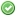rfc:array_column

## Introduction

This RFC proposes a new array function that returns the values of the specified column from a multi-dimensional array. Inspired by database methods like `PDOStatement::fetchColumn()`, `array_column()` moves useful functionality into the core that once had to be implemented in userland code with sometimes complex loops.

This has been submitted as a pull request on GitHub, where there has already been a significant amount of discussion.

## Specification

### Description

`array array_column(array \$input, mixed \$columnKey[, mixed \$indexKey])`

`array_column()` returns the values from a single column of the input array, identified by the columnKey. Optionally, you may provide an indexKey to index the values in the returned array by the values from the indexKey column in the input array.

### Parameters

input

A multi-dimensional array (record set) from which to pull a column of values.

columnKey

The column of values to return. This value may be the integer key of the column you wish to retrieve, or it may be the string key name for an associative array.

indexKey

(Optional.) The column to use as the index/keys for the returned array. This value may be the integer key of the column, or it may be the string key name.

### Return Values

Returns an array of values representing a single column from the input array.

### Examples

#### Example #1: Get column of first names from recordset

```<?php
// Array representing a possible record set returned from a database
\$records = array(
array(
'id' => 2135,
'first_name' => 'John',
'last_name' => 'Doe'
),
array(
'id' => 3245,
'first_name' => 'Sally',
'last_name' => 'Smith'
),
array(
'id' => 5342,
'first_name' => 'Jane',
'last_name' => 'Jones'
),
array(
'id' => 5623,
'first_name' => 'Peter',
'last_name' => 'Doe'
)
);

\$firstNames = array_column(\$records, 'first_name');
print_r(\$firstNames);```

The above example will output:

```Array
(
 => John
 => Sally
 => Jane
 => Peter
)```

#### Example #2: Retrieve a column of values from a numerically-indexed array

```<?php
\$records = array(
array(1, 'John', 'Doe'),
array(2, 'Sally', 'Smith'),
array(3, 'Jane', 'Jones')
);

\$lastNames = array_column(\$records, 2);
print_r(\$lastNames);```

The above example will output:

```Array
(
 => Doe
 => Smith
 => Jones
)```

#### Example #3: Get column of last names from recordset, indexed by the "id" column

```<?php
// Using the \$records array from Example #1
\$lastNames = array_column(\$records, 'last_name', 'id');
print_r(\$lastNames);```

The above example will output:

```Array
(
 => Doe
 => Smith
 => Jones
 => Doe
)```

#### Example #4: Mismatched columns

With `array_column()` the relationship in finding the values of columnKey to indexKey is much like that of a SQL left join. All values of the columnKey are returned. When a corresponding indexKey cannot be found, the value will be keyed with an integer, starting from zero.

The following examples will all use the same \$mismatchedColumns array defined here:

```<?php
\$mismatchedColumns = array(
array(
'a' => 'foo',
'b' => 'bar',
'e' => 'baz'
),
array(
'a' => 'qux',
'c' => 'quux',
'd' => 'corge'
),
array(
'a' => 'grault',
'b' => 'garply',
'e' => 'waldo'
),
);```

In this example, all rows contain an “a” key, but only two contain a “b” key. If we want to retrieve all “a” values and key them by “b” values, then `array_column()` behaves like this:

```<?php
\$foo = array_column(\$mismatchedColumns, 'a', 'b');
\$bar = array('bar' => 'foo', 'qux', 'garply' => 'grault');

/*
Both \$foo and \$bar contain values that look like this:

Array
(
[bar] => foo
 => qux
[garply] => grault
)
*/```

However, if we want to retrieve all “b” values and key them by “a” values, we will only have two elements in the resulting array, since only two rows contain “b” values.

```// There is a corresponding "a" value for each "b" value
print_r(array_column(\$mismatchedColumns, 'b', 'a'));

/*
Array
(
[foo] => bar
[grault] => garply
)
*/

// There are no corresponding "c" values for either "b" value
print_r(array_column(\$mismatchedColumns, 'b', 'c'));

/*
Array
(
 => bar
 => garply
)
*/```

#### Example #5: indexKey Collisions

In the event that more than one row contains the same value for indexKey, then the last columnKey value for that indexKey will overwrite the previous value.

```// Using the \$records array from Example #1
\$firstNames = array_column(\$records, 'first_name', 'last_name');
print_r(\$firstNames);

/*
Array
(
[Doe] => Peter
[Smith] => Sally
[Jones] => Jane
)
*/```

## Proposal and Patch

The patch (including tests) for this proposal is available in GitHub Pull Request #257.

## Mailing list discussion

The mailing list discussion is available here.

## Voting

Voting ends not before Friday, January 18, 2013. The PHP language is not changed, so a 50% + 1 majority is required.

Accept array_column() for inclusion in PHP?
Real name Yes No
ab (ab)aharvey (aharvey)arpad (arpad)ashnazg (ashnazg)blanchonvincent (blanchonvincent)cataphract (cataphract)chx (chx)davey (davey)derick (derick)fa (fa)frozenfire (frozenfire)googleguy (googleguy)guilhermeblanco (guilhermeblanco)gwynne (gwynne)hradtke (hradtke)ifeghali (ifeghali)juliens (juliens)jwage (jwage)kalle (kalle)kriscraig (kriscraig)levim (levim)lstrojny (lstrojny)marco (marco)mfonda (mfonda)mike (mike)mj (mj)nikic (nikic)oaass (oaass)pajoye (pajoye)patrickallaert (patrickallaert)peehaa (peehaa)pollita (pollita)ralphschindler (ralphschindler)rasmus (rasmus)rdohms (rdohms)remi (remi)salathe (salathe)seld (seld)shiflett (shiflett)stas (stas)treffynnon (treffynnon)tyrael (tyrael)willfitch (willfitch)zeev (zeev)Final result: 38 6
This poll has been closed.

## Changelog

• 1.0 (2012-06-21): Initial draft, following discussion on on GitHub Pull Request #56
• 2.0 (2013-01-11): Updated to reflect mailing list and pull request feedback.